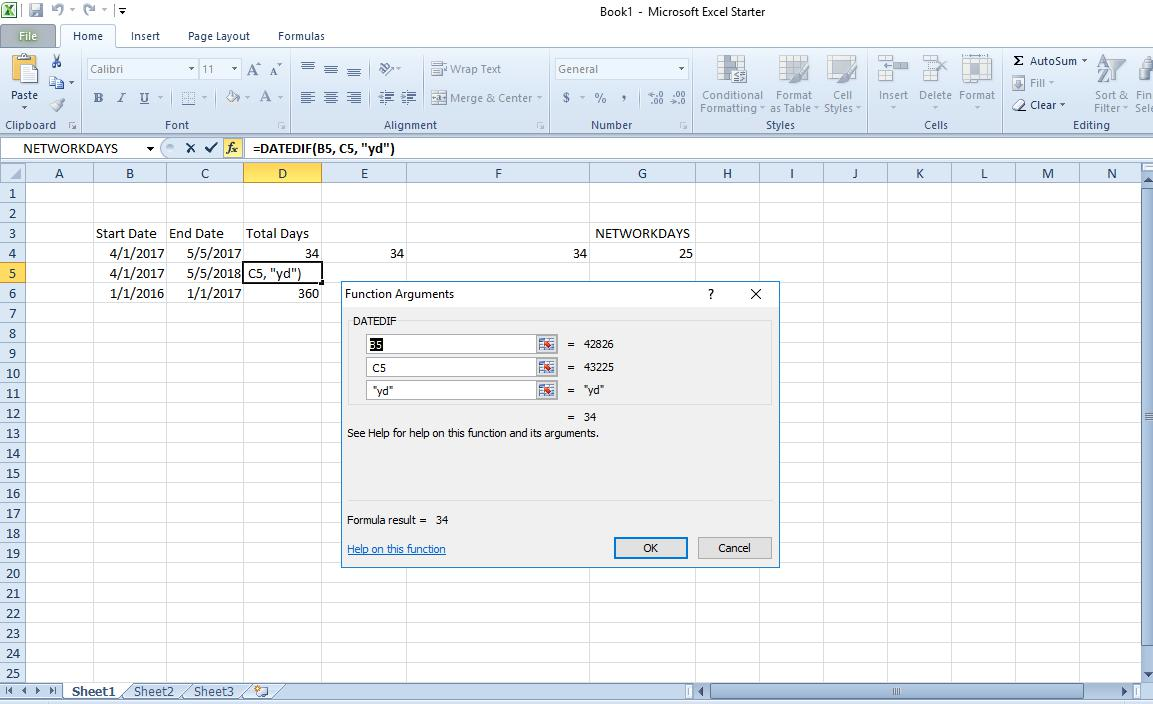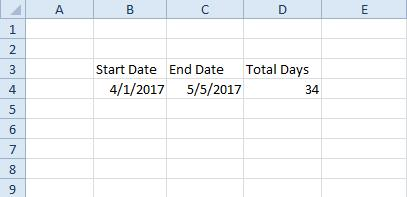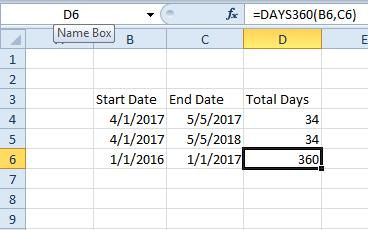# How To Calculate Days Between Two Dates in Excel

Many Excel users will need to add start and end date columns to spreadsheets. As such, Excel includes a few functions that tell you how many days there are between two separate dates. DATEDIF, DAYS360, DATE and NETWORKDAYS are four functions you can add to cells that will tell you the number of days between two dates. This is how you find the number of days between dates with and without those functions in Excel.### How to Find the Difference Between dates without a Function

First, you can find the difference between dates by subtracting them. Excel doesn’t include a subtract function, but you can still add subtraction formulas to cells. So open a blank Excel spreadsheet, and enter a start and end date in cells B4 and C4 as in the snapshot below. Note that the dates should be in U.S. format with month first, day second and year third.In the example above, the dates are 4/1/2017 and 5/5/2017. Now you should select cell D4 and click inside the function bar at the top of the sheet. Input ‘=C4-B4’ in the bar and press Enter. Cell D4 will return a value of 34 if you entered the same dates in the cells as in the snapshot above. As such, there are 34 days between April 1, 2017, and May 5, 2017.

### The DATE Function

Alternatively, you can find the difference between two dates without with the DATE function. Then you can find the number of days by entering the dates in the function bar instead of spreadsheet cells. The basic syntax for that function is: =DATE(yyyy, m, d)-DATE(yyyy, m, d); so you input the dates backwards.

Let’s add that function with the same 4/1/2017 and 5/5/2017 dates. Select a cell on the spreadsheet to add the function to. Then click inside the function bar, input ‘=DATE(2017, 5, 5)-DATE(2017, 4, 1)’ and press Enter. You might also need to select a general number format for the cell if the value returned is in date format. With a general number format, the cell will return a value of 34 days as shown in the snapshot below.### The DATEDIF Function

DATEDIF is a flexible function you can calculate the total days between dates with by both entering dates on the spreadsheet or in the function bar. However, DATEDIF is not listed on Excel’s Insert Function window. Thus, you’ll need to enter it directly in the function bar. The syntax of the DATEDIF function is: DATEDIF(start_date, end_date, unit). You can enter a start date and end date or cell references to specific dates in the function, and then add the unit days to the end of it.

So select a cell to add DATEDIF to on the spreadsheet, and then click in the formula bar to input the function. To find the difference between the two dates you entered in cells C4 and B4, input the following in the function bar: ‘=DATEDIF(B4, C4, “d”).’ The DATEDIF cell will include the number of days between the dates as shown directly below.However, this is much more flexible than the DATE function as you can modify the units. For example, suppose you needed to count the number the days between two dates, but also ignore the years. You can do that by adding ‘yd’ to the function. For example, enter ‘4/1/2017’ and ‘5/5/2018’ in two cells, and then include ‘yd’ in the function as shown below.That returns a value of 34 days between 4/1/2017 and 5/5/2018, which is right if you ignore the year. If the function didn’t ignore the year, the value would be 399.

### The DAYS360 Function

The DAYS360 function is one that finds the total days between dates based on a 360-day calendar, which is more widely adopted for financial years. As such, that might be a better function for account spreadsheets. It won’t make much difference for dates just a few months apart, but for longer periods DAYS360 will return slightly different values than the other functions.

Enter ‘1/1/2016’ and ‘1/1/2017’ in cells B6 and C6 on your spreadsheet. Then click a cell to include the DAYS360 function in, and press the fx button beside the function bar. Select DAYS360 to open the window shown directly below.Press the Start_date button and select cell B6. Then click the End_date button and select cell C6 on the spreadsheet. Press OK to add DAYS360 to the spreadsheet, which will return a value of 360.### The NETWORKDAYS Function

What if you need to find the difference between two dates, but exclude weekends from the equation? DATEDIF, DATE and DAYS360 aren’t going to be much good for such a scenario. NETWORKDAYS is a function that finds the number of days between dates without including any weekends, and it can also factor in extra holidays, such as bank holidays. So it’s supposed to be a function for project planning. The function’s basic syntax is: =NETWORKDAYS(start_date, end_date, [holidays]).

To add NETWORKDAYS to a spreadsheet, click a cell for the function and press the fx button. Select NETWORKDAYS to open its window in the snapshot directly below. Thereafter, click the Start_date button and select a cell on your spreadsheet that includes a start date. Press the End_date button, select a cell with an end date in it and press OK to add the function to spreadsheet.In the screenshot directly above, the start and end dates are 4/1/2017 and 5/5/2017. The NETWORKDAYS function returns a value of 25 days between the dates without the weekends. With the weekends included, the total days is 34 as with earlier examples.

To include extra holiday days in the function, enter those dates in additional spreadsheet cells. Then press the Holidays cell reference button on the NETWORKDAYS function window and select the cell, or cells, that include the holiday dates. That will deduct the holidays from the final figure.

So there are numerous ways you can calculate days between start and end dates in Excel spreadsheets. More recent Excel versions also include a DAYS function that you can find the difference between a couple of dates with. Those functions will certainly come in handy for spreadsheets that include lots of dates.

## One thought on “How To Calculate Days Between Two Dates in Excel”Ab says:
How many days are there 1/16/2019 through 2/26/2019
The “Days” function returns 41 but if you count I think it’s 42

Disclaimer: Some pages on this site may include an affiliate link. This does not effect our editorial in any way.

Todays Highlights